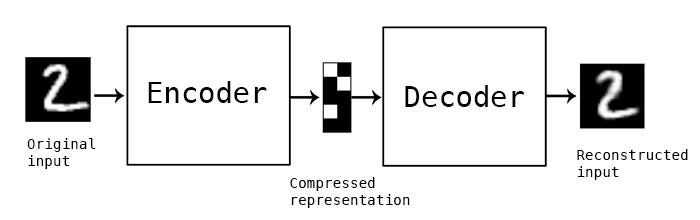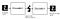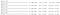source: https://blog.keras.io/building-autoencoders-in-keras.html

# Autoencoders in Computer Vision

## An implementation with Python

In my previous article, I’ve been talking about Generative Adversarial Networks, a class of algorithms used in Deep Learning which belong to the category of generative models.

## Buinding an Autoencoder

To build an AE, we need three components: an encoder network which compresses the image, a decoder network which decompresses it, and a distance metric which can evaluate the similarity between the original and generated image. The whole model is trained to find weights for both the inner networks which minimizes the loss (=distance metric).source: https://blog.keras.io/building-autoencoders-in-keras.html
`from keras.datasets import mnistimport numpy as np(x_train, _), (x_test, _) = mnist.load_data()x_train = x_train.astype('float32') / 255.x_test = x_test.astype('float32') / 255.x_train = x_train.reshape((len(x_train), np.prod(x_train.shape[1:])))x_test = x_test.reshape((len(x_test), np.prod(x_test.shape[1:])))print(x_train.shape) #let's have a look at the shapeprint(x_test.shape)`
`import matplotlib.pyplot as pltplt.imshow(x_test.reshape(28, 28))plt.gray()`
`import kerasfrom keras import layersencoding_dim = 24  # 24 floats, it means that we have a compression factor of 784 (image input shape)/ 24 = 32.7input_img = keras.Input(shape=(784,))encoded = layers.Dense(encoding_dim, activation='relu')(input_img)decoded = layers.Dense(784, activation='sigmoid')(encoded)autoencoder = keras.Model(input_img, decoded)encoder = keras.Model(input_img, encoded)encoded_input = keras.Input(shape=(encoding_dim,))decoder_layer = autoencoder.layers[-1]decoder = keras.Model(encoded_input, decoder_layer(encoded_input))autoencoder.compile(optimizer='adam', loss='binary_crossentropy') #per-pixel binary crossentropy loss`
`autoencoder.fit(x_train, x_train,                epochs=50,                batch_size=256,                shuffle=True,                validation_data=(x_test, x_test))encoded_imgs = encoder.predict(x_test)decoded_imgs = decoder.predict(encoded_imgs)`…….
`plt.figure(figsize=(9, 4))plt.subplot(121)plt.imshow(x_test.reshape(28, 28))plt.gray()plt.subplot(122)plt.imshow(decoded_imgs.reshape(28, 28))plt.gray()`

## Conclusions

In the context of image generation, in recent years GANs have outperformed the AEs methods. However, AEs are not only employed for image generation, nor are they employed solely in Computer vision field. Indeed, there is a variety of fields of interest:

• Anomaly detection → because of the way it works, AEs are trained to precisely reproduce the most frequent characteristics of the original data. As such, whenever there are anomalies in the original data, the reconstruction performances are worse than average, which should be an indicator of potential anomalies.
• Image processing → in addition to image generation, AEs have also other applications in the computer vision field. Namely, the capability of denoising an image in order to improve the quality of images and movie that are poor in conditions. Similar to this latter, and paired with super-resolution, AEs are used also in the clinical field to improve the quality of X-Ray images, so that they can be further used for image segmentation or object detection tasks.

Cloud Specialist at @Microsoft | MSc in Data Science | Machine Learning, Statistics and Running enthusiast

## More from Valentina Alto

Cloud Specialist at @Microsoft | MSc in Data Science | Machine Learning, Statistics and Running enthusiast